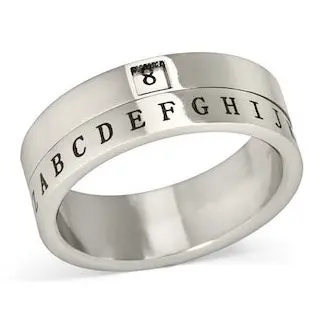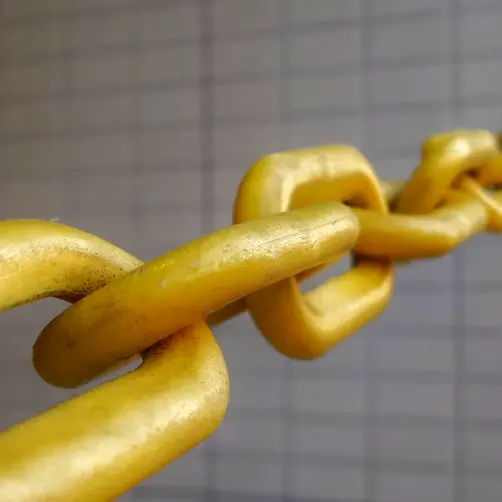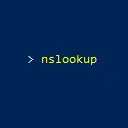# Binary Converter

This binary converter allows you to convert numbers between different bases.

from
to

## More Tools

### Encoder/DecoderEncode or decode using URL encoding, Base64 encoding, or Base64URL encoding.

### Security ToolsCheck up on the security of your website or domain. Validate your SSL/TLS certificate.

### DNS ToolsConvert numbers between binary, octal, decimal, and hexadecimal representations.

### Subnet CalculatorUse the subnet calculator to calculate the network details of an IPv4 subnet. Use an IP address or CIDR block notation.

### Binary

An important part of your computer is called "binary." You interact with your computer every day, but you might not know that binary numbers make everything work under the hood. From clicking your mouse all the way down to executing instructions in memory and storing data onto disk drives, binary is at the heart. Binary is the language of 0s and 1s that a computer can understand.

There are various ways to convert numbers between different bases. For example, you can convert base 2 (binary), octal (base 8), decimal (base 10), and hexadecimal (base 16) numbers. These calculations require more work than you might expect and are prone to errors as well! Fortunately, you can use a binary number converter to make the calculations easy.

Every IP address in an IPv4 subnet can be represented in its dotted-decimal notation as well as its binary equivalent. For example, the IP address 10.0.5.0 (base 10) is 00001010 00000000 00000101 00000000 (base 2). Subnet masks can also be represented in dotted-decimal notation or the binary equivalent.

### How to Convert Decimal to Binary

To convert a number from decimal to binary:

1. Divide the number by 2 and write down the quotient and remainder.
2. Continue dividing each quotient by 2 until you reach 0.
3. Write down each remainder in reverse order to get the number in binary.

Example (decimal number 21):

Divide by 2 Quotient Remainder
21 / 2 10 1
10 / 2 5 0
5 / 2 2 1
2 / 2 1 0
1 / 2 0 1
Now write the remainders in reverse order to get the binary number: 10101.

### Binary Converter and Free APIs

The binary converter is a tool that converts numbers between different bases: decimal to binary, binary to decimal, octal to binary, and so on.

Use this binary converter tool to convert numbers between different bases. Simply enter the number to convert, select the bases to convert from and to, and click "Convert".

View the results in the free API to see how you can integrate the converter into your own software projects.